The attached file, dax-30-2006-2015, contains the closing levels of the German stock index, DAX-30, and the daily percentage changes for the period between February 2006 and the end of 2015. Develop ARIMA/GARCH models for the observed data.

The analysis should include

1. a detailed explanation of the steps taken to arrive at the final model;
2. an examination of the residuals to check its whiteness;
3. forecasts for the index for ten days beyond the end of data set; and
4. your qualitative evaluation of the models.

The model I arrived at for describing and forecasting the DAX stock market is ARIMA(0,1,0) (with a Box-Cox transformation) + GARCH(2,1). Below are the requested explanations and additional calculations.

# 1. Steps for arriving at the model.

dax <- read.csv("dax30dailyreturns-2006-2015.csv")

The first step is to look at the data. For simplicity, we are going to treat the daily data as a basic time series, ignoring actual dates as well as the gaps in the data caused by weekends.

daxTs <- ts(dax$price) autoplot(daxTs)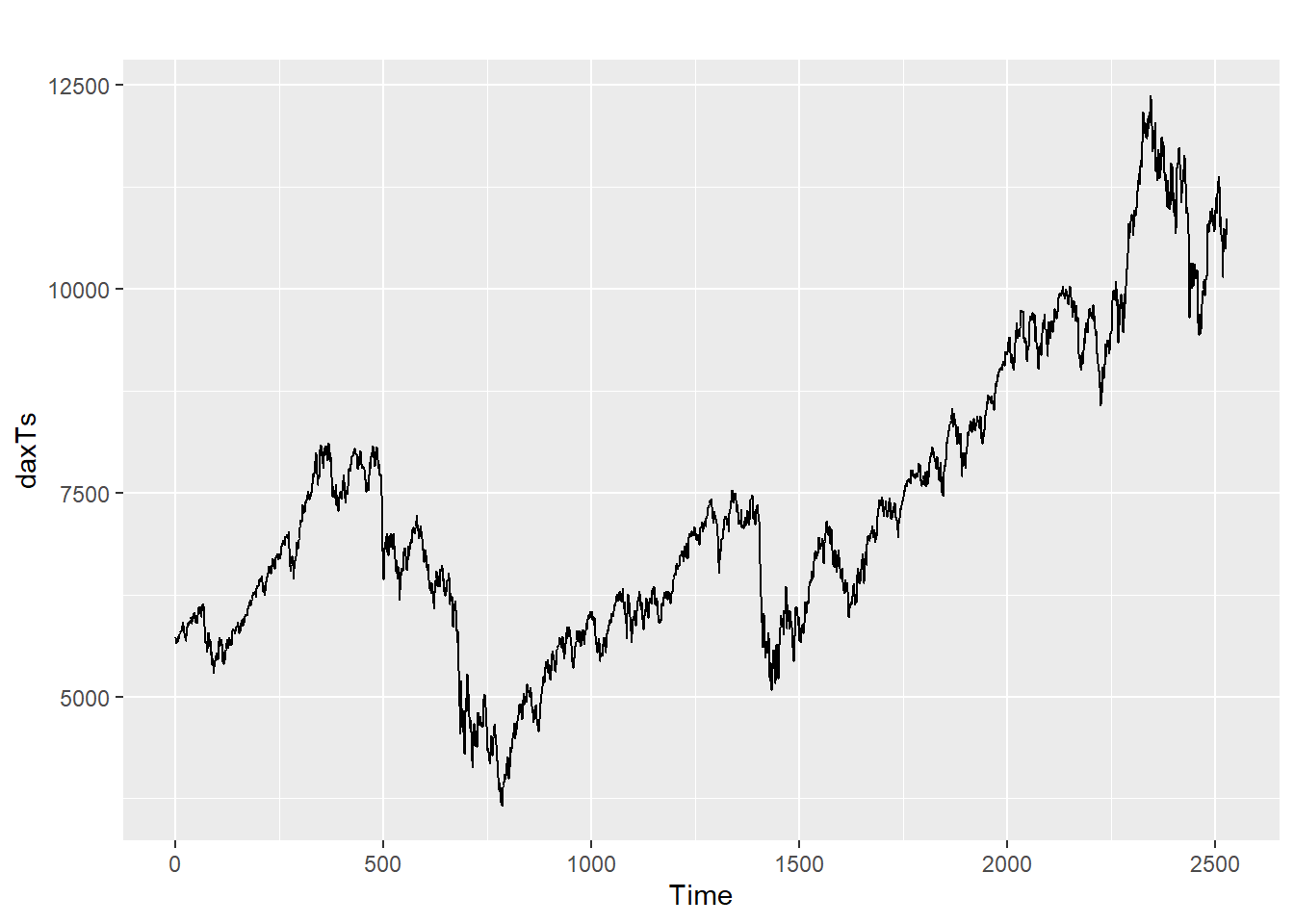Next we will run auto.arima to find two things: First, what the suggested arima model is, and also we will check the residuals for signs of volatility clustering, or conditional volatility. The suggested model is ARIMA(0,1,0) random walk without drift, with a BoxCox transformation with a lambda of 0.86. The unconditional variance is 803.1. Checking residuals shows that the plot is close to white noise - the ACF is mostly below threshold levels. However, the residuals plot does appear to show clusters of volatility. daxAr <- daxTs %>% auto.arima(allowdrift = T, lambda="auto") daxAr ## Series: . ## ARIMA(0,1,0) ## Box Cox transformation: lambda= 0.8602771 ## ## sigma^2 = 803.1: log likelihood = -12036.52 ## AIC=24075.04 AICc=24075.04 BIC=24080.87 checkresiduals(daxAr)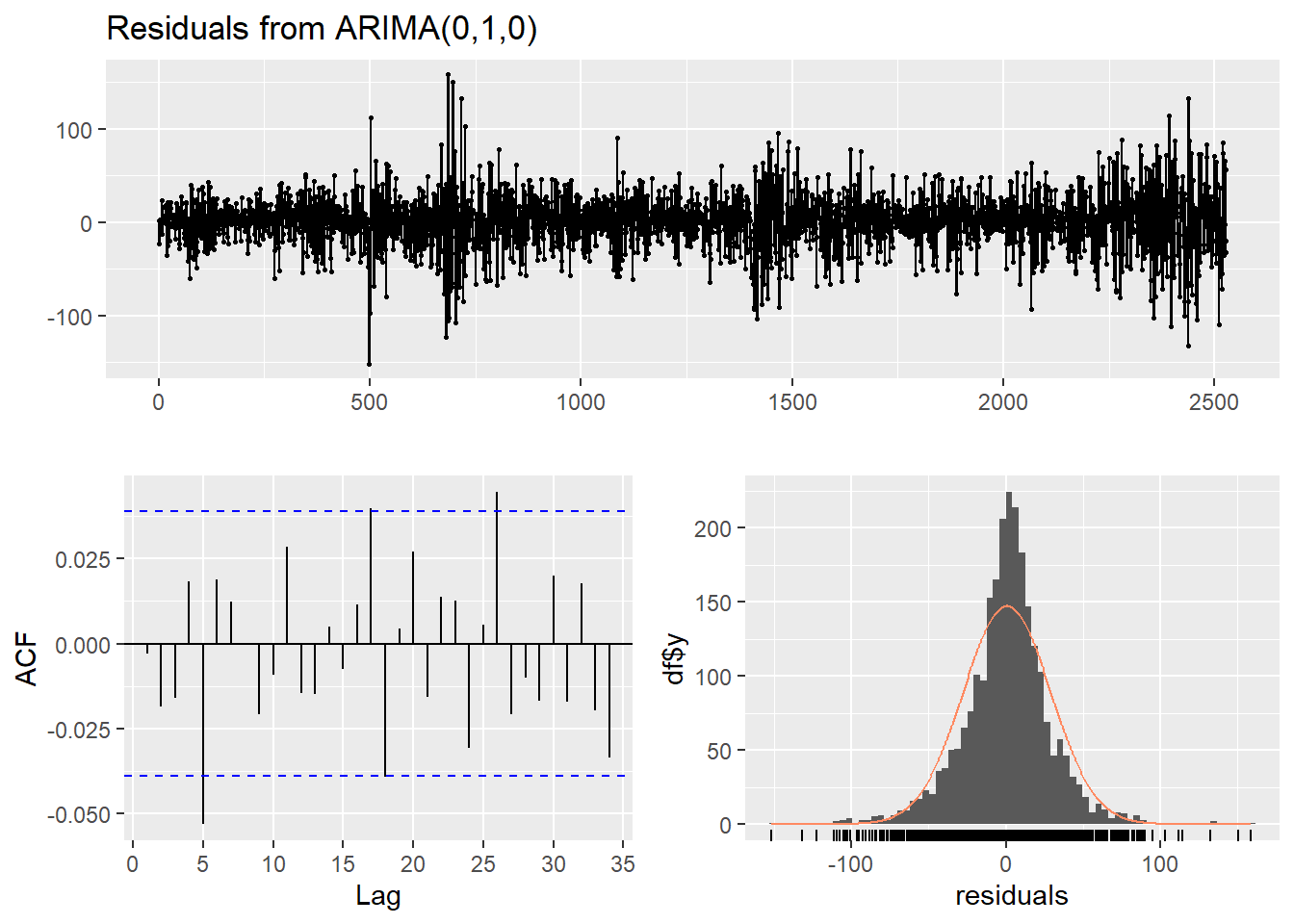## ## Ljung-Box test ## ## data: Residuals from ARIMA(0,1,0) ## Q* = 12.202, df = 10, p-value = 0.2718 ## ## Model df: 0. Total lags used: 10 To check more closely for residuals clustering we can plot the square of residuals. This seems to clearly show that volatility is clustered - in other words our unconditional variance term is likely not accurate. plot(daxAr$residuals^2)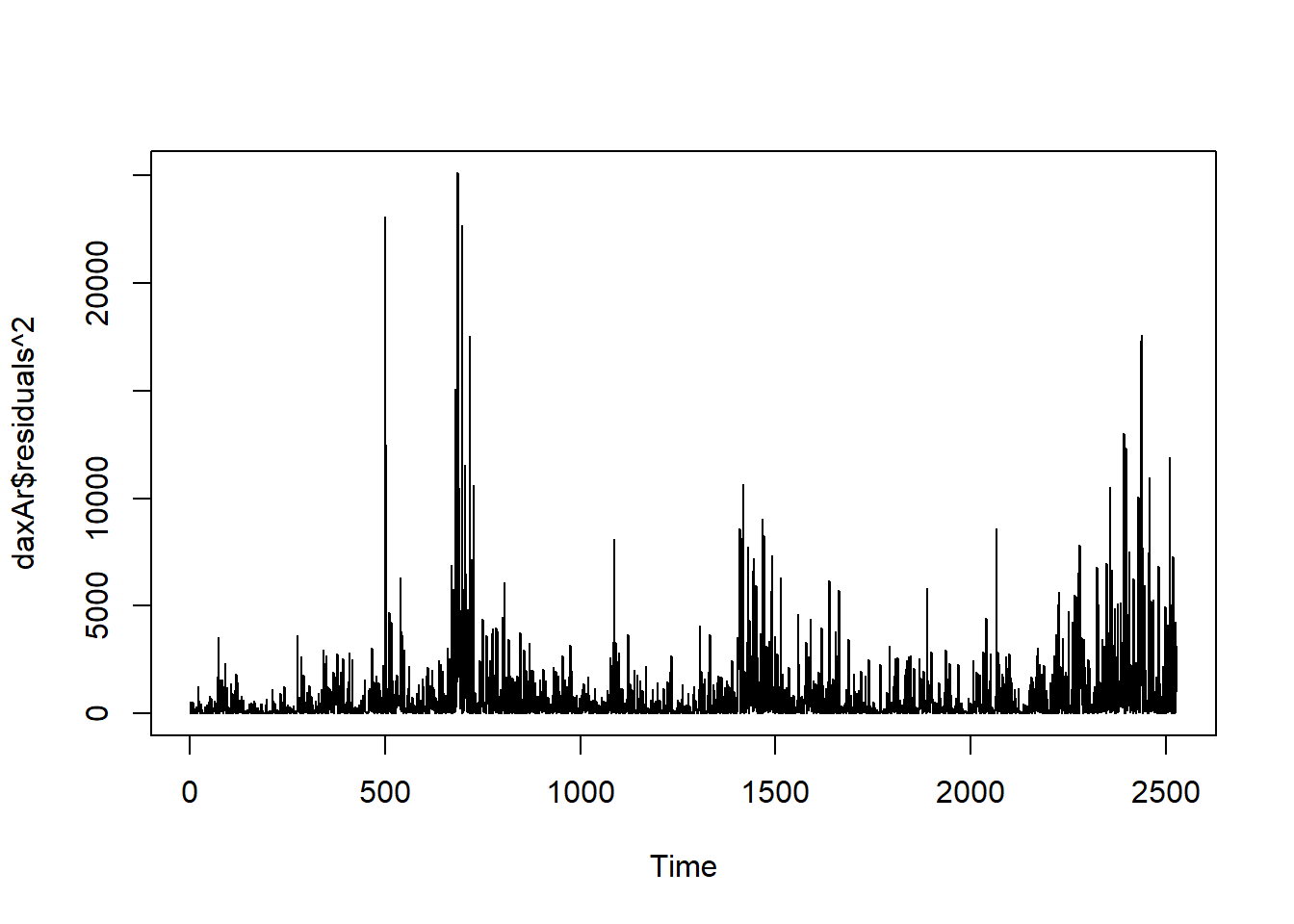To deal with conditional volatility, we will model the data using the GARCH model. Because R does not provide a function to automatically pick parameters for the GARCH(p,q) model, the loop function below will test values of 1-3 for both p and q. The formula assumes an ARMA(0,0) (which applies no autoregression or moving average model) on an already differenced and BoxCox transformed DAX dataset.

Below, GARCH(2,1) is shown to provide the best fit, with the lowest AIC of 9.305.

daxDiff = daxTs %>% BoxCox(daxAr$lambda) %>% diff() for (p in 1:3){ for (q in 1:3){ formula_str <- sprintf("arma(0,0) + garch(%s, %s)", p, q) %>% reformulate() daxFit <- garchFit(formula = formula_str, data = daxDiff , trace = F) str <- sprintf("GARCH(%s, %s) - AIC: %s", p, q, daxFit@fit$ics["AIC"])
print(str)
}
}
##  "GARCH(1, 1) - AIC: 9.30612738370554"
##  "GARCH(1, 2) - AIC: 9.31151287056042"
## Warning in sqrt(diag(fit$cvar)): NaNs produced ##  "GARCH(1, 3) - AIC: 9.30812298993742" ##  "GARCH(2, 1) - AIC: 9.30479578848344" ##  "GARCH(2, 2) - AIC: 9.30558724079916" ##  "GARCH(2, 3) - AIC: 9.30634996672897" ##  "GARCH(3, 1) - AIC: 9.30575733159481" ##  "GARCH(3, 2) - AIC: 9.30586520535771" ## Warning in sqrt(diag(fit$cvar)): NaNs produced
##  "GARCH(3, 3) - AIC: 9.30567511667776"

Now we will assess the results from the ARMA(0,0) + GARCH(2,1). It is worth noting that GARCH(2,1) may be slightly overkill, because as mentioned in class, the “simple GARCH (1,1) models often works very well in representing volatility clustering occurring in financial markets where conditional variance is positively correlated.”

GARCH is applied to an ARMA model which does not include differencing, so I will run the garchFit function on already differenced data.

daxGarch = garchFit(~arma(0,0)+garch(2,1),  data    =   daxDiff ,   trace   =   F)
summary(daxGarch)
##
## Title:
##  GARCH Modelling
##
## Call:
##  garchFit(formula = ~arma(0, 0) + garch(2, 1), data = daxDiff,
##     trace = F)
##
## Mean and Variance Equation:
##  data ~ arma(0, 0) + garch(2, 1)
## <environment: 0x000001ffafe60a80>
##  [data = daxDiff]
##
## Conditional Distribution:
##  norm
##
## Coefficient(s):
##        mu      omega     alpha1     alpha2      beta1
##  1.536901  13.858488   0.037866   0.061404   0.885926
##
## Std. Errors:
##  based on Hessian
##
## Error Analysis:
##         Estimate  Std. Error  t value Pr(>|t|)
## mu       1.53690     0.44395    3.462 0.000536 ***
## omega   13.85849     3.62296    3.825 0.000131 ***
## alpha1   0.03787     0.01960    1.932 0.053352 .
## alpha2   0.06140     0.02415    2.542 0.011008 *
## beta1    0.88593     0.01569   56.465  < 2e-16 ***
## ---
## Signif. codes:  0 '***' 0.001 '**' 0.01 '*' 0.05 '.' 0.1 ' ' 1
##
## Log Likelihood:
##  -11751.61    normalized:  -4.650419
##
## Description:
##  Sat Dec 10 20:24:27 2022 by user: gideo
##
##
## Standardised Residuals Tests:
##                                 Statistic p-Value
##  Jarque-Bera Test   R    Chi^2  205.9121  0
##  Shapiro-Wilk Test  R    W      0.9878889 7.891146e-14
##  Ljung-Box Test     R    Q(10)  6.416474  0.7791441
##  Ljung-Box Test     R    Q(15)  9.068859  0.8738896
##  Ljung-Box Test     R    Q(20)  14.32942  0.8134087
##  Ljung-Box Test     R^2  Q(10)  4.147413  0.940452
##  Ljung-Box Test     R^2  Q(15)  8.986804  0.8782064
##  Ljung-Box Test     R^2  Q(20)  15.4441   0.7504572
##  LM Arch Test       R    TR^2   6.977546  0.8590938
##
## Information Criterion Statistics:
##      AIC      BIC      SIC     HQIC
## 9.304796 9.316341 9.304788 9.308985

# 2. Examination of whiteness

Using this GARCH model we will assess some plots to check performance. Note that the residuals of the GARCH model are the same as the the differenced series - GARCH affects the variance, not the estimated value.

ACF The below ACF plot shows that most lag correlations do not exceed the 95% confidence interval correlation band, although some do by a little bit. The 5th lag correlation is most concerning. Although not shown for space reasons, the PACF chart shows similar levels of partial autocorrelations, also with a slightly concerning 5th lag.

ggAcf(daxGarch@residuals)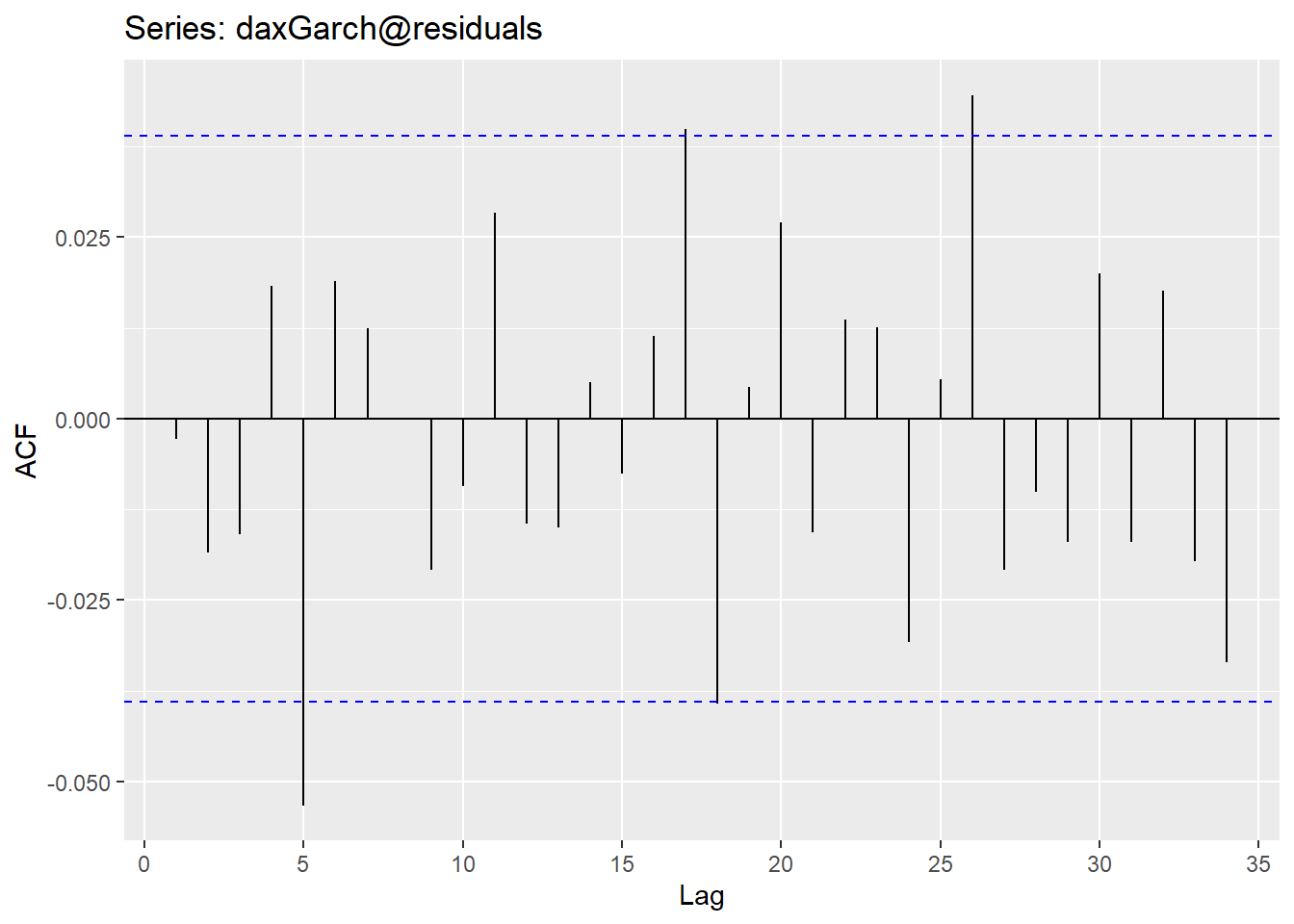Ljung-Box test The large p-value of the Ljung Box (0.89) test suggest that the residuals are white noise.

Box.test(daxGarch@residuals)
##
##  Box-Pierce test
##
## data:  daxGarch@residuals
## X-squared = 0.020138, df = 1, p-value = 0.8872

QQPlot This plot shows some lack of normality at tail ends. Although it is useful to know that that the distribution of residuals is not perfectly normal, this result does not necessarily mean that the residuals are not white noise.

residuals(daxGarch) %>% qqnorm()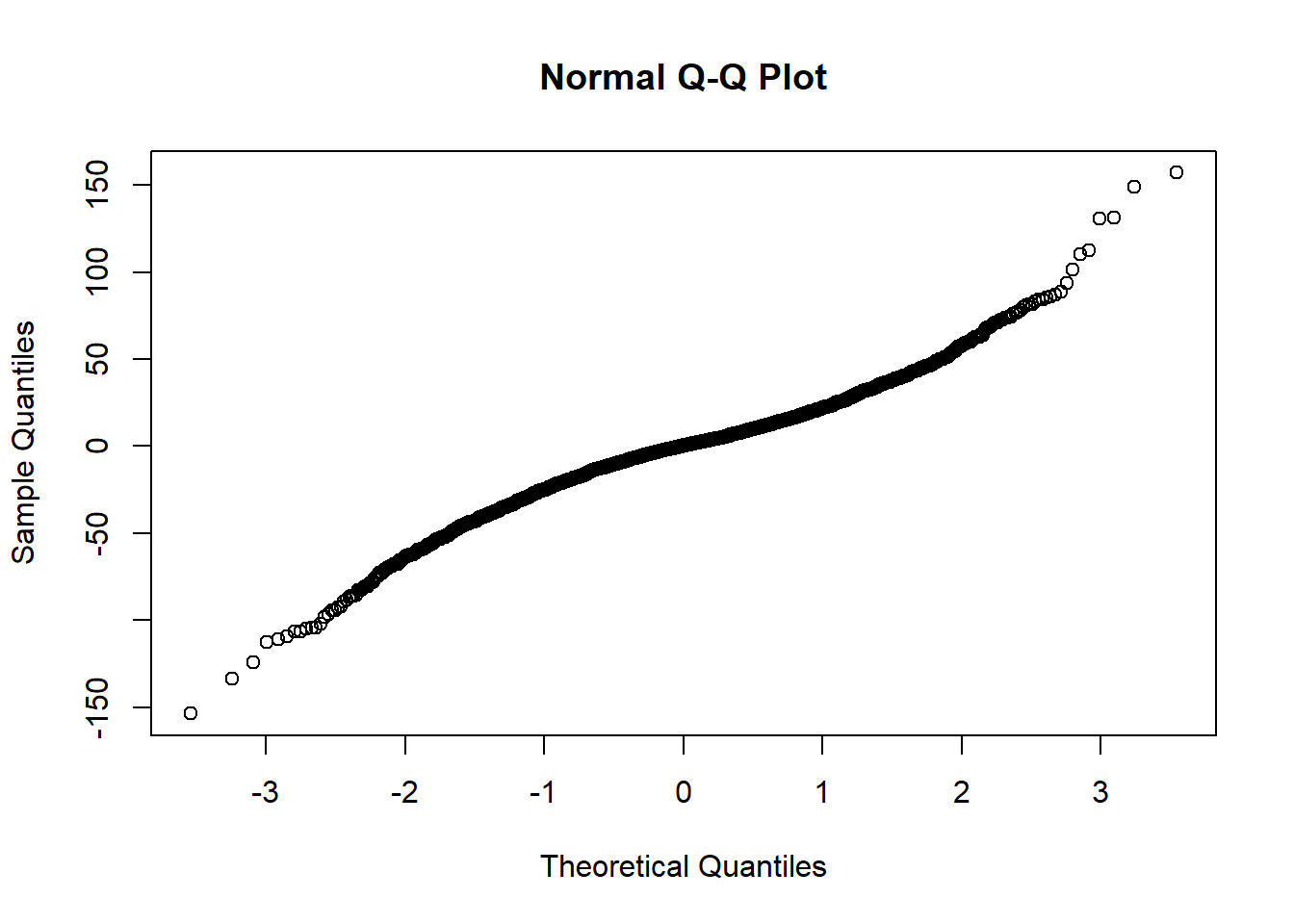# 3. Forecasting

Using the “predict” function we can predict differenced data for 10 days into the future. A plot and tabular data is below. The plot shows the confidence interval gradually converging to +/- 55, which is 1.96 times the series’ unconditional standard deviation of 28.3, which was given to us by the original ARIMA function.

predict(daxGarch, plot=TRUE, nx=50)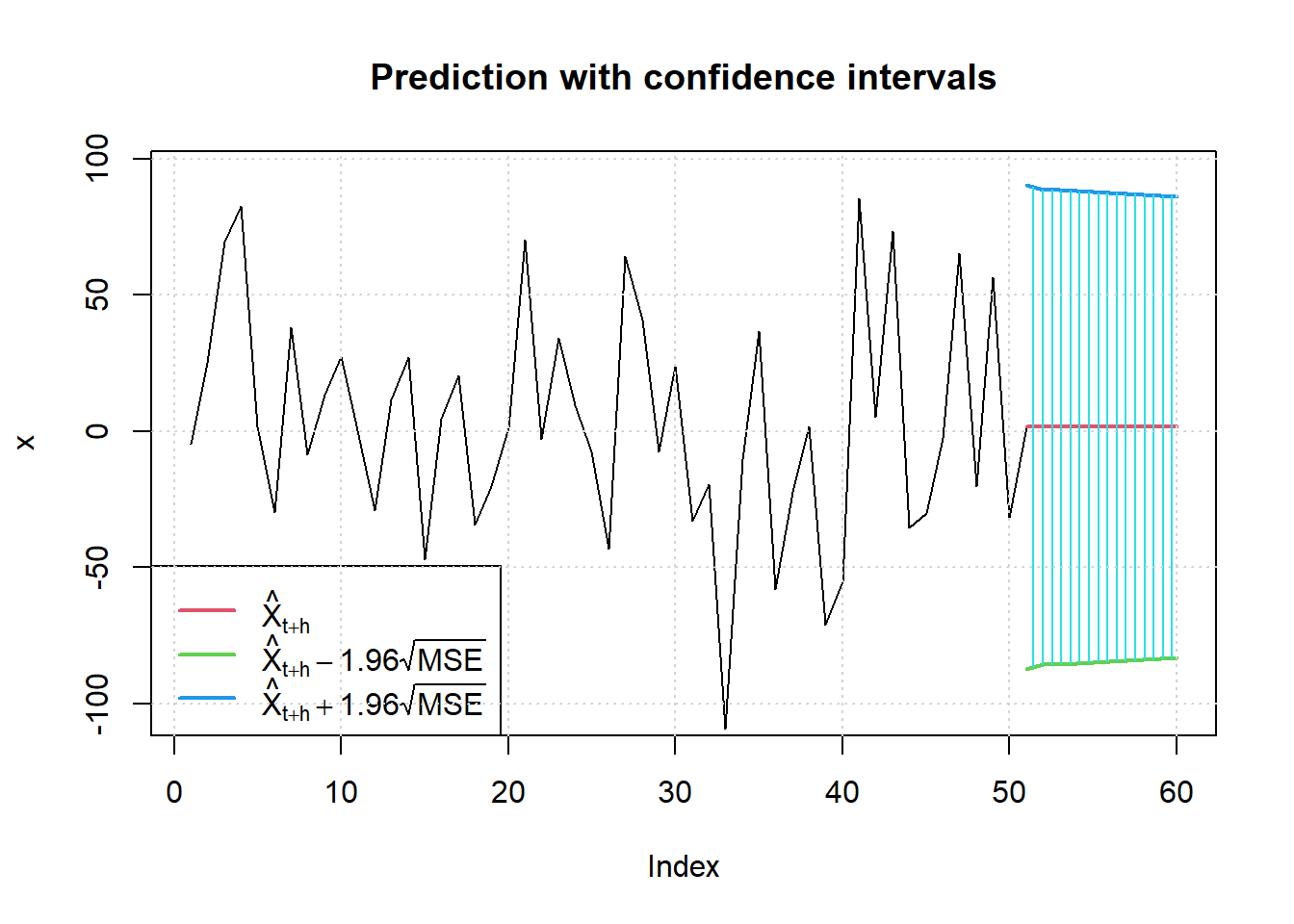##    meanForecast meanError standardDeviation lowerInterval upperInterval
## 1      1.536901  45.34574          45.34574     -87.33912      90.41292
## 2      1.536901  44.52459          44.52459     -85.72968      88.80349
## 3      1.536901  44.40135          44.40135     -85.48814      88.56195
## 4      1.536901  44.23601          44.23601     -85.16408      88.23788
## 5      1.536901  44.07508          44.07508     -84.84868      87.92248
## 6      1.536901  43.91565          43.91565     -84.53620      87.61000
## 7      1.536901  43.75789          43.75789     -84.22699      87.30079
## 8      1.536901  43.60176          43.60176     -83.92098      86.99479
## 9      1.536901  43.44726          43.44726     -83.61817      86.69197
## 10     1.536901  43.29438          43.29438     -83.31852      86.39232

Note that these are predictions for the differenced series, or the change in each unit of time. To see the actual series prediction (without a 95% confidence interval) we can reverse the BoxCox and differencing transformations and then add these values to the final point of the DAX series.

predict(daxGarch)$meanForecast %>% diffinv() %>% InvBoxCox(lambda = daxAr$lambda) + daxTs[length(daxTs)]
##   10744.01 10745.67 10747.51 10749.45 10751.49 10753.59 10755.76 10757.98
##   10760.24 10762.55 10764.90

# 4. Qualitative examination

First we should just describe what our model does, after the series has been appropriately transformed via Box-Cox and then differenced.

The ARMA(0,0) + GARCH(2,1) model (with differencing) describes a univariate time series where:

• ARMA(0,0) describes a random walk (for an already-differenced series).
• GARCH(2,1) describes a generalized ARCH model with p=2 and q=1, capturing the conditional volatility of the time series.

The below plot of volatility from the GARCH model alongside the original data demonstrates that the model used correctly assumes higher volatility during apparent volatility clusters.

par(mfrow = c(2,1))
plot(diff(daxTs))
plot(volatility(daxGarch), type="l")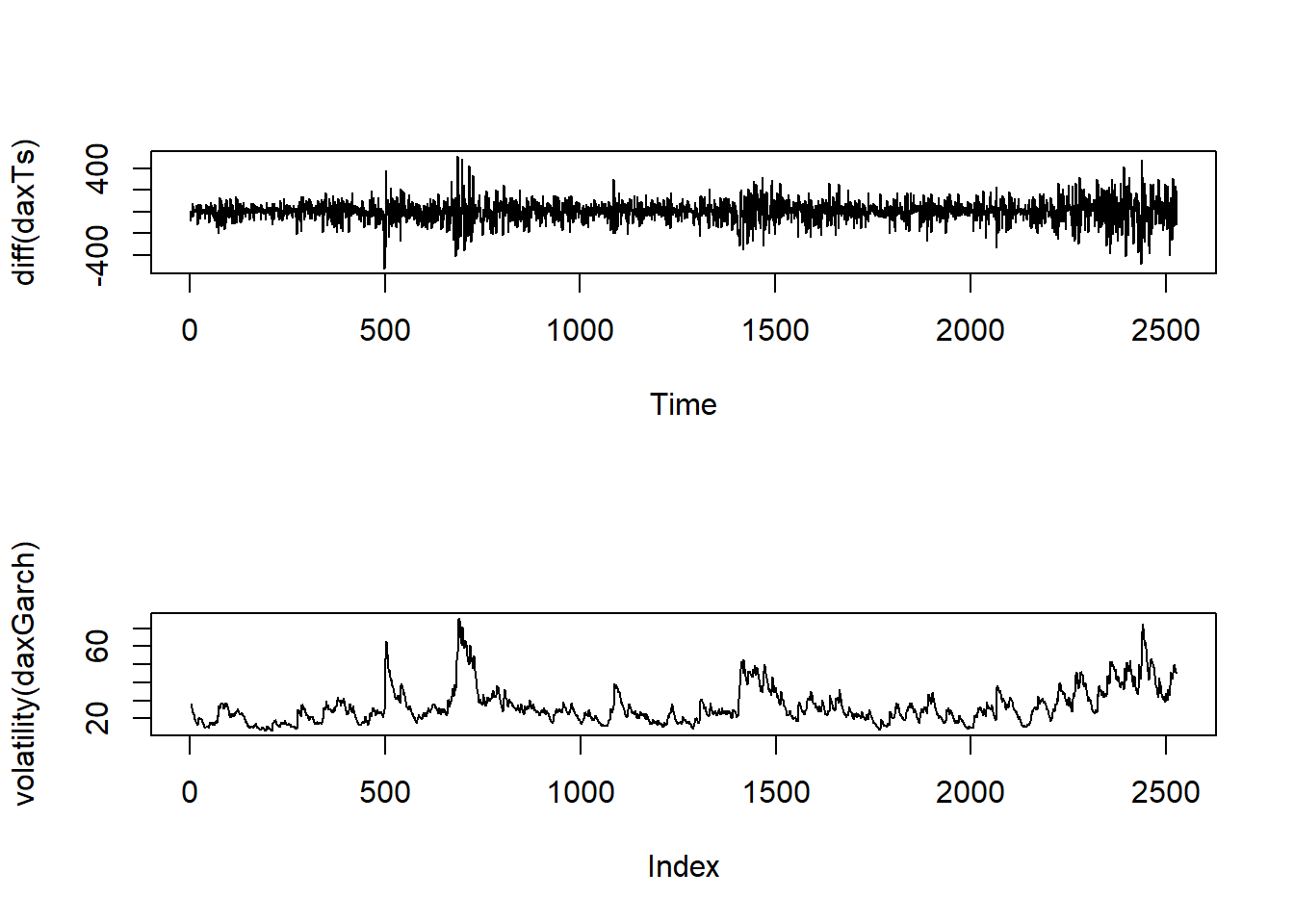The period of highest volatility (x-index between 500 and 1,000) aligns with the onset of a global recession in 2008-2009, which makes sense. The reason having conditional volatility is important for financial time series is that volatility changes based on economic and political conditions.

The high volatility at the end of the series is also shown by the wide confidence interval in the prediction plot, which then converges toward the unconditional variance.

Finally, here is the plot of daxGarch, which shows that most points fall withing the 95th confidence interval of the prediction. The biggest “miss” is the enormous drop at time period 500. Although such a sudden fall would be hard to capture in any model, this does point at one of the flaws in the standard GARCH model - which is that it does not allow for different reactions to negative or positive changes. In reality, large negative changes tend to create more volatility than large positive changes. 1

plot(daxGarch, which = 3)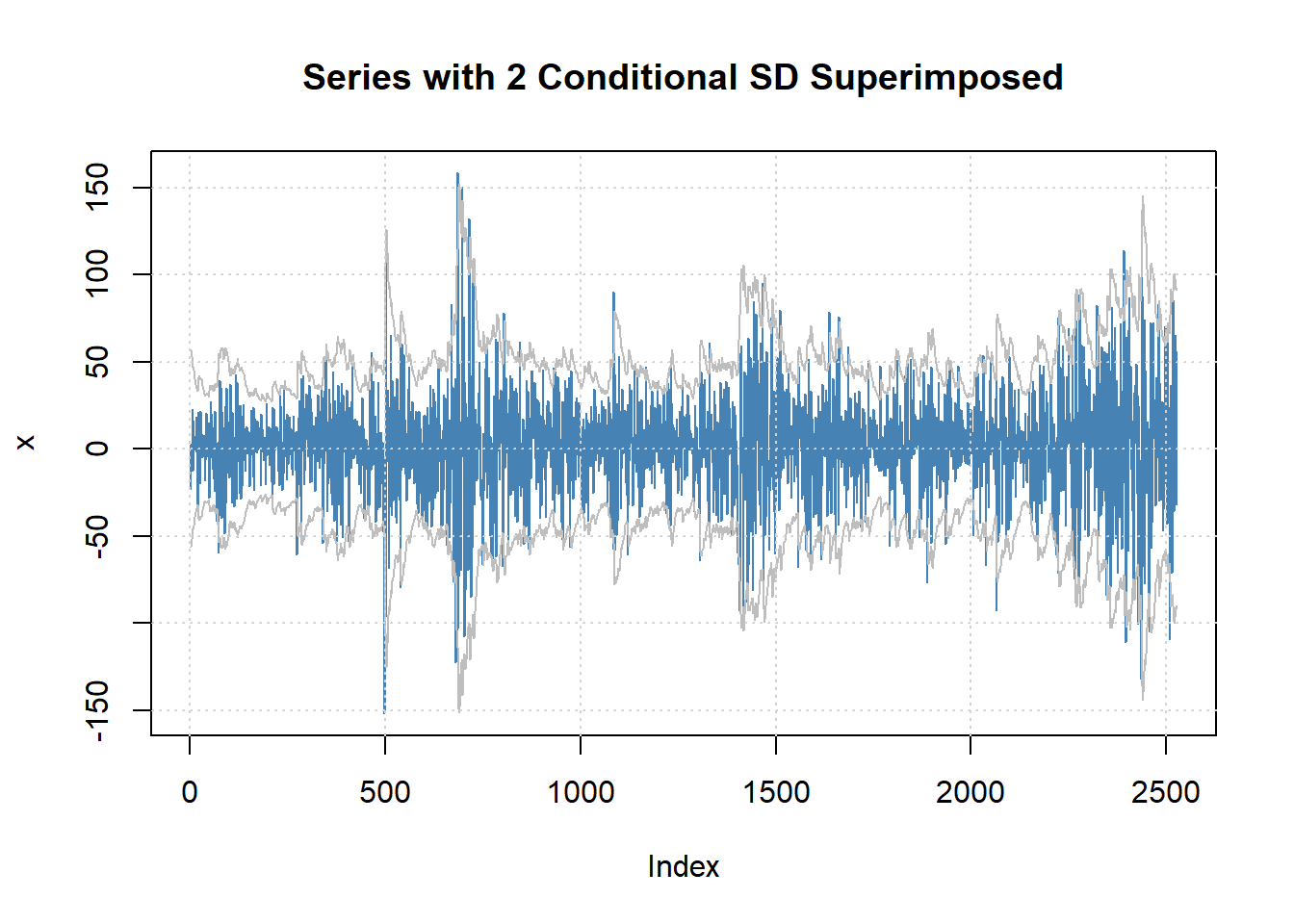Based on this analysis, I would say that our model:

• correctly performs transformations with a first-order differencing and Box-Cox transformation resulting in white noise.
• correctly refrains from applying an autoregression or moving average model, based on the ACF and PACF analyses above.
• identifies conditional variance accurately. However, the model could perhaps be improved by updating to an asymmetric GARCH model, which could have performed better at periods of negative shocks. Examples of this include the TGARCH and AGARCH models. 2